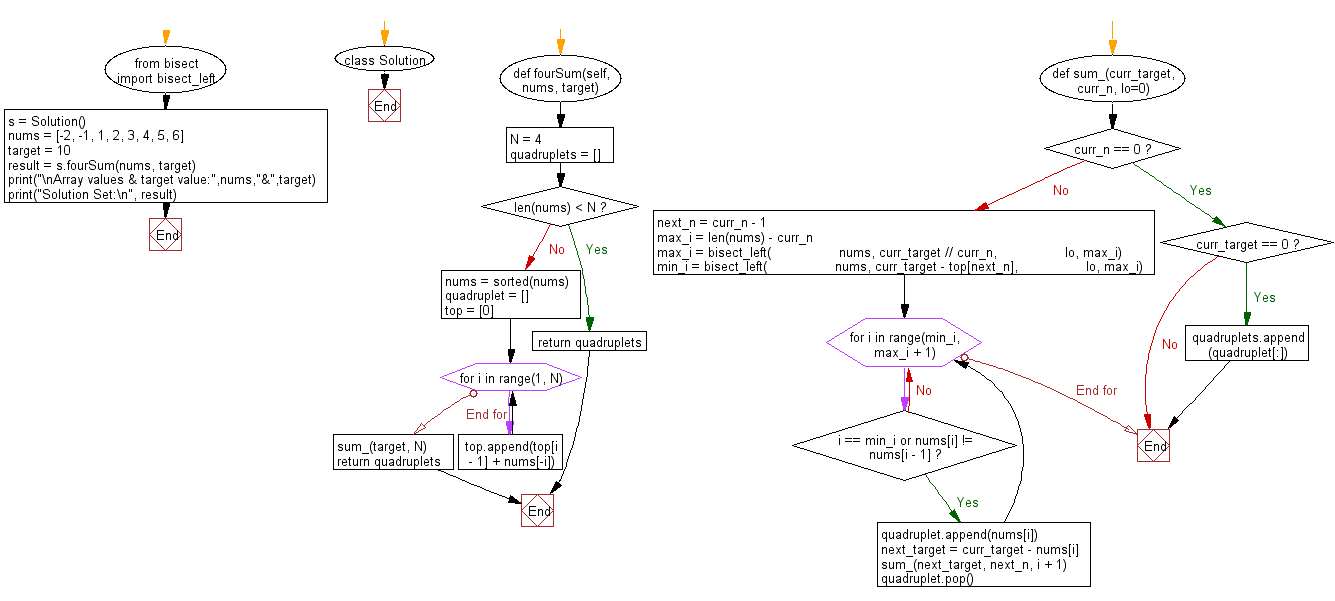﻿ Python Bisect: Find four elements from a given array of integers whose sum is equal to a given number - w3resource# Python Bisect: Find four elements from a given array of integers whose sum is equal to a given number

## Python Bisect: Exercise-9 with Solution

Write a Python program to find four elements from a given array of integers whose sum is equal to a given number. The solution set must not contain duplicate quadruplets.

Sample Solution:

Python Code:

``````#Source: https://bit.ly/2SSoyhf
from bisect import bisect_left
class Solution:
def fourSum(self, nums, target):
"""
:type nums: List[int]
:type target: int
:rtype: List[List[int]]
"""
N = 4
if len(nums) < N:
nums = sorted(nums)

# Let top[i] be the sum of largest i numbers.
top = 
for i in range(1, N):
top.append(top[i - 1] + nums[-i])

# Find range of the least number in curr_n (0,...,N)
# numbers that sum up to curr_target, then find range
# of 2nd least number and so on by recursion.
def sum_(curr_target, curr_n, lo=0):
if curr_n == 0:
if curr_target == 0:
return

next_n = curr_n - 1
max_i = len(nums) - curr_n
max_i = bisect_left(
nums, curr_target // curr_n,
lo, max_i)
min_i = bisect_left(
nums, curr_target - top[next_n],
lo, max_i)

for i in range(min_i, max_i + 1):
if i == min_i or nums[i] != nums[i - 1]:
next_target = curr_target - nums[i]
sum_(next_target, next_n, i + 1)

sum_(target, N)

s = Solution()
nums = [-2, -1, 1, 2, 3, 4, 5, 6]
target = 10
result = s.fourSum(nums, target)
print("\nArray values & target value:",nums,"&",target)
print("Solution Set:\n", result)
```
```

Sample Output:

```Array values & target value: [-2, -1, 1, 2, 3, 4, 5, 6] & 10
Solution Set:
[[-2, 1, 5, 6], [-2, 2, 4, 6], [-2, 3, 4, 5], [-1, 1, 4, 6], [-1, 2, 3, 6], [-1, 2, 4, 5], [1, 2, 3, 4]]
```

Flowchart:## Visualize Python code execution:

The following tool visualize what the computer is doing step-by-step as it executes the said program:

Python Code Editor:

What is the difficulty level of this exercise?

Test your Programming skills with w3resource's quiz.

﻿

## Python: Tips of the Day

How to make a chain of function decorators?

```from functools import wraps

def makebold(fn):
@wraps(fn)
def wrapped(*args, **kwargs):
return "<b>" + fn(*args, **kwargs) + "</b>"
return wrapped

def makeitalic(fn):
@wraps(fn)
def wrapped(*args, **kwargs):
return "<i>" + fn(*args, **kwargs) + "</i>"
return wrapped

@makebold
@makeitalic
def hello():
return "hello world"

@makebold
@makeitalic
def log(s):
return s

print hello()        # returns "<b><i>hello world</i></b>"
print hello.__name__ # with functools.wraps() this returns "hello"
print log('hello')   # returns "<b><i>hello</i></b>"
```

Ref: https://bit.ly/3cVz5iw